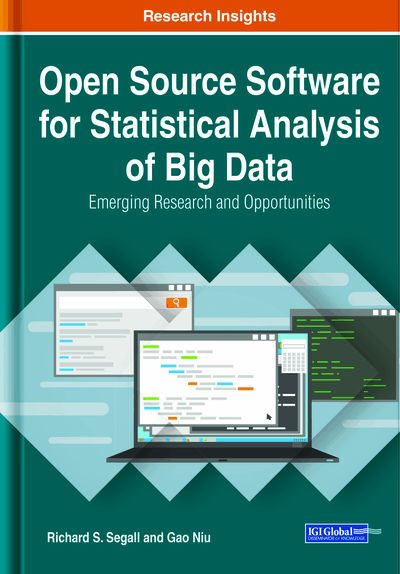# Generalized Linear Model for Automobile Fatality Rate Prediction in R

Gao Niu, Alan Olinsky
DOI: 10.4018/978-1-7998-2768-9.ch005
OnDemand:
(Individual Chapters)
Available
\$37.50
No Current Special Offers

## Abstract

This chapter demonstrates the descriptive and statistical modeling function in R. The automobile fatal accident data of the United States is extracted from the Fatality Analysis Reporting System (FARS). The model will be used to understand significant contributing factors of automobile accident death when a fatal crash happens. First, descriptive analysis is performed by basic R functions and packages. Then, generalized linear model (GLM) with logit link function is explored and constructed. Finally, multiple validation metrics are introduced and calculated to ensure the reasonability and accuracy of the predictions. The focus of this chapter is to demonstrate the power and flexibility of the most popular Open Source Statistical Software (OSSS) through a real data analysis.
Chapter Preview
Top

## R Working Environment

R’s working environment screenshot is attached by Figure 1. It is made very simple by focusing on the core calculation engine behind the scene. There are three sub-windows demonstrated in the following example. R console on the left demonstrates the direct numerical, as well as systematic messages. R editor on the upper right is where programmer’s syntax can be organized, saved, and executed. The window with a Histogram graph is an active window and it will be triggered when a graphical function is executed.

Table 1 lists out all of the programmed functions in the tool bar based on R version 3.6.1 published on July 5th 2019.

## Key Terms in this Chapter

Variable Grouping: It is a process of group variables levels, so that the variable is more statistically significant and interpretable in the model construction. Variable grouping includes continuous variable categorization and discrete variable recategorization.

GIGO: Garbage in, garbage out. It is an acronym of ineffective control of input data, model selection and output results, that model produces unrecognizable result.

80/20 Validation: 80/20 validation is a type of model validation. 80% of data is used for model construction, and 20% of data is used for model validation.

Interaction Term: It is the effect change on dependent variables when multiple independent variables exist at the same time. It is represented as a product of two or more independent variables in the regression equation

K-Fold Cross Validation: K-fold cross validation is a type of model validation. K-fold cross validation first partition dataset into k sections evenly. Each fold is considered as holdout section once and rest is used for model construction. After all holdout section are predicted, actual and predicted dependent quantities are compared for validation.

75/25 Validation: 75/25 validation is a type of model validation. 75% of data is used for model construction, and 25% of data is used for model validation.

Holdout Sample: It is a portion of data that is separated out from training data and model construction process. Holdout data is used for testing and model validation.

Backward Selection: Backward selection is a type of stepwise variable selection which starts with the model with all candidate variables. Each variable will be in turn eliminated and the model with the most statistical criteria deterioration will be subtracted. The process is repeated until no more variable subtraction could improve the statistical criteria.

Forward Selection: Forward selection is a type of stepwise variable selection which starts with the model with no variable. All variables will be tested and the one variable with the most statistical criteria improvement will be added. The process is repeated until no more variable addition could improve the statistical criteria.

Calendar Year Validation: Calendar year validation is a type of model validation which uses data from a certain period of time to analyze, interpret and construct model, then a different holdout period of time data is tested and validated.

Stepwise Variable Selection: Stepwise variable selection process is method of fitting generalized linear models in which variables are added or subtracted one at a time from the set of variables based on predefined criteria.

## Complete Chapter List

Search this Book:
Reset